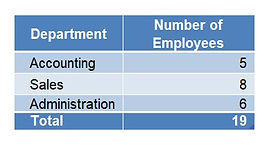top of page

# Microsoft Excel COUNTIF FunctionUse COUNTIF to conditionally count a range of values or text, based on a single criterion

Would you like to know how many employees work in a particular department? Or how many sales were made by a specific rep? Or perhaps how many sales came in over or under target?  COUNTIF will quickly and easily accomplish this!

In the example below, we count the number of employees by department.

Step 1. Set up your data correctly, preferably converting it to a table.Step 2. Add the COUNTIF formula to the Number of Employees column for the results in the table below.DETAILED EXPLANATION

SYNTAX:  =COUNTIF(Range in which Criteria is found ,Criteria)

EXAMPLE 1: Formula example if referring to a table range (tables are preferable, as will expand/contract as add/remove data):

=COUNTIF(tblEmployeeData[Department],[@Department])

EXAMPLE 2: Formula example if referring to a regular data range:

=COUNTIF(\$D\$4:\$D\$22,E27)               OR               =COUNTIF(\$D\$4:\$D\$22,"Accounting")

Give the COUNTIF function a try, and let us know if you have any questions, or would like to arrange training on this

or other Excel functions and features!

© 2018 DJH Training & Application Solutions Inc.

bottom of page